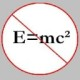You are currently browsing the tag archive for the ‘integration bee’ tag.

Okay, I thought earlier that part 3 of the above series of posts would be my last one. For some reason, this series has turned out to be a somewhat popular one when considering the fact that a big chunk of blog visitors visit it. Probably, this is due to the fact that the 2008 MIT Integration Bee is going to be held sometime soon – I have no idea exactly when – or perhaps, there are other Integration Bees that are going to be held in other colleges/universities sometime soon. If I get some more feedback/interest, then I will consider posting more results/identities/tricks on this same topic.

As you might have noticed from the title of the post, this identity isn’t related to definite integrals; it is related to indefinite integrals. Of course, since definite integrals form a “subset” of indefinite integrals, we can apply this identity to either one of them.

Let me begin by posing two problems, which I ask you to solve in your head. If you are able to do so, then you probably know the trick that is stated below, and hence you may stop reading this post; else, continue reading.

Problem 1: Evaluate$\displaystyle \int e^x (\frac{x-2}{x^3}) \, dx$.

Problem 2: Evaluate$\displaystyle \int e^x (\ln x + \frac1{x}) \, dx$.

And, here’s our identity.$(4) \displaystyle \int e^x (f(x) + f'(x)) \, dx = e^x f(x) + C$,

where$C$ is the constant of integration.

Proof: We use integration by parts. Recall,$\displaystyle \int u\, dv = uv - \int v\, du$, where$u \equiv f(x)$ and$v \equiv g(x)$. So, if we let$\displaystyle v = e^x$, then we have$\displaystyle \int e^x f(x) \, dx = \int f(x) \, d(e^x) = f(x) e^x - \int e^x d(f'(x))$, which leads us to our identity.

Now, you should be able to solve the above problems in your head in just a couple of seconds if not less.

Solution 1:$\displaystyle e^x/x^2 + C$.

Solution 2:$e^x \ln x + C$.

If you have found this particular series of posts useful, drop me a comment. Doing so will provide me the motivation to post more stuff on this topic in the near future.

Here are some challenging integrals to evaluate.$(1)$ Evaluate$\displaystyle \int \sin(101x)\sin^{99}x \, dx$. (MIT Integration Bee)$(2)$ Evaluate$\displaystyle \int^{1}_{0}\frac {\arctan x}{x + 1} \, dx$.$(3)$ For$n > 1$, prove that$\displaystyle \int^{\infty}_{0}\frac {dx}{\left(x + \sqrt {1 + x^{2}}\right)^{n}} = \frac {n}{n^{2} - 1}$.$(4)$ If$f$ is a bounded non-negative function, then show that$\displaystyle \int_{0}^{\infty}f(x + \frac {1}{x})\frac {\ln(x)}{x}dx = 0$.$(5)$ Evaluate$\displaystyle \int_{0}^{1}\ln(\sqrt {1 - x} + \sqrt {1 + x})dx$.$(6)$ Evaluate$\displaystyle \int_{ - \pi /2}^{\pi /2} \frac1{2007^{x} + 1}\cdot \frac {\sin^{2008}x}{\sin^{2008}x + \cos^{2008}x} \, dx$.

The problems above aren’t necessarily in increasing order of difficulty; however, the last one can be almost impossible to evaluate if one doesn’t know the right “trick”, which will be the subject of my third identity in my series of posts titled, A few useful identities related to definite integrals, which you can find in the Problem Corner.

Note: Most of the problems (posted above) have been borrowed from people who posted the same in AoPS.

### Blog Stats

• 346,162 hitsprof dr drd horia or… on My first postprof drd horia orasa… on My first postprof dr mircea orasa… on Inequality with lognotedscholar on Self-referential Paradoxes, In…prof dr mircea orasa… on Inequality with logprof dr mircea orasa… on Inequality with logprof dr mircea orasa… on 2010 in reviewkenji on Basic category theory, Iprof dr mircea orasa… on Solution to POW-10: Another ha…prof drd horia orasa… on Continued fraction for eprof drd horia orasa… on Inequality with logprof dr mircea orasa… on Solution to POW-12: A graph co…prof dr mircea orasa… on The Character of Physical…prof dr mircea orasa… on Milk and Tea puzzleprof drd horia orasa… on Inequality with log